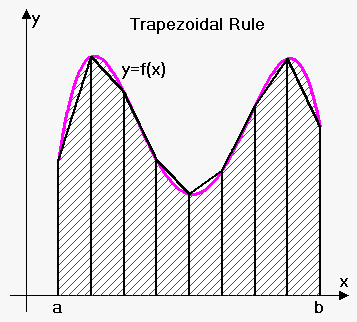# Trapezoidal Rule

## Related calculators: Riemann Sum Calculator for a Function , Trapezoidal Rule Calculator for a Function

We will obtain Trapezoidal rule (approximation) from averaging Right and Left Endpoint approximations: int_a^bf(x)dx~~1/2(L_n+R_n)=1/2(sum_(i=1)^nf(x_(i-1))Delta x+sum_(i=1)^nf(x_i)Delta x) .

Rewriting it we obtain Trapezoidal Rule: int_a^bf(x)dx~~T_n=(Delta x)/2(f(x_0)+2f(x_1)+2f(x_2)+...+2f(x_(n-1))+f(x_n)).It is called Trapezoidal because as appeared this formula gives approximation by approximating area not with rectangles but trapezoids, with height of trapezoid Delta x and lengths of bases f(x_(i-1)) and f(x_i) . Thus area of i-th trapezoid is Delta x((f(x_(i-1))+f(x_i))/2). Adding all trapezoids will give trapezoidal rule.

It is worth noting that if function is concave up then trapezoidal rule overestimates integral, if function is concave down then trapezoidal rule underestimates integral.

Example 1. Use Trapezoidal Rule to approximate value of int_1^2 1/x^2dx with n=5.

Here a=1, b=2, f(x)=1/x^2 and n=5. So, Delta x=(b-a)/n=(2-1)/5=0.2.

So, int_1^2 1/x^2(dx)~~T_n=0.2/2 (f(1)+2f(1.2)+2f(1.4)+2f(1.6)+2f(1.8)+f(2))=

=0.1(1/1^2+2/(1.2)^2+2/(1.4)^2+2/(1.6)^2+2/(1.8)^2+1/2^2)~~0.505783.

In Left Endpoint, Right Endpoint note we found for this example L_n=0.580783 and R_n=0.430783.

So, 1/2(L_n+R_n)=1/2(0.580783+0.430783)=0.505783=T_n as expected.

True value of integral is I=int_1^2 1/x^2dx=0.5.

So, Trapezoidal Rule overestimates integral as expected (function f(x)=1/x^2 is concave up on [1,2]).

When we approximate integral we will always have some error: E=int_a^bf(x)dx-App where App is approximation and E is error.

Error Bound for Trapezoidal Rule. Suppose |f''(x)|<=M for a<=x<=b then |E|<=(M(b-a)^3)/(12n^2).

Example 2. How large should we take n in order to guarantee that the Trapezoidal Rule approximation for int_1^2 1/x^2 dx are accurate to within 0.0002?

Here a=1, b=2, f(x)=1/x^2.

Then f'(x)=-2/x^3 and f''(x)=6/x^4.

Therefore |f''(x)|<=6 for 1<=x<=2.

Thus, (6(2-1)^3)/(12n^2)>0.0002 or n^2>1/(0.0004).

So, n>1/sqrt(0.0004)=50.

So, we need to take n=51.